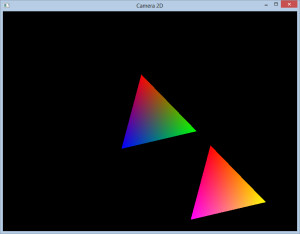# OpenGL Camera 2d with zoom and rotation

I’ve been working on OpenGL for some time now and one of the things that some people have asked me is how to do that 2d camera with zoom and rotation in a similar way to a post regarding a XNA 2D Camera I wrote some time ago.
Some of them where just subtracting the camera position to all objects drawn, but that’s just ‘ugly’ in my opinion. And the advantage of using a more complicated (at first glance) system as a matrix is that you can easily apply more complicated operations like scaling and rotation.The Camera2D class is simple enough:

```class Camera2d { public: Camera2d(void); virtual ~Camera2d(void); inline void set_zoom(const float zoom){ _zoom = zoom; } inline float get_zoom()const{ return _zoom; } Matrix get_transformation(); protected: void recreate_tranformation_matrix(); protected: // Saves Last Property // This is used for knowing when to update the Matrix struct Transformation { Matrix _matrix; Vector2f _last_pos; float _last_zoom; Vector2f _last_origin; float _last_rotation; void update(const Vector2f& p, const Vector2f& origin, const float zoom, const float rotation) { _last_pos = p; _last_origin = origin; _last_zoom = zoom; _last_rotation = rotation; } }; protected: float _zoom; Transformation _transform; public: Vector2f _pos; Vector2f _origin; float _rotation; };```

As the name implies Vector2f and Matrix are the Vector and the Matrix, the full code is included in the project that you can download at the end of the article so I’m just going to mention the most important parts.
In Camera2D class the most important function is the recreate_tranformation_matrix(), get_transformation() just checks if we should recreate it.

```  void Camera2d::recreate_tranformation_matrix() { // Update Variables _transform.update(_pos,_origin,_zoom,_rotation);   _transform._matrix = /* Create our camera matrix, translation to origin, then rotation, scale and finally translation to the camera position */ Matrix::CreateTranslation(-_pos.X,-_pos.Y, 0) * Matrix::CreateScale(_zoom, _zoom, 1)* Matrix::CreateRotationZ(_rotation) * Matrix::CreateTranslation(_origin.X,_origin.Y, 0); }```

As you can see we create our finally Matrix considering several things, first there’s the camera origin or center. This is useful for rotations and scales when you want to keep the camera focusing on a certain element, usually the center. The most common positions will probably be the center of the camera or 0,0.
Next we have a rotation on Z, next Zoom, this is how much the camera will zoom in or out, again the origin will impact where the zoom center is. Finally the camera position itself. The order of these operations is extremely important since mat1*mat2 != mat2*mat1.

So how could you use this?
First the camera setup

```// Create our new camera Camera2d* camera = new Camera2d(); camera->_origin.set(width/2.0f, height/2.0f); // width and height are the screen sizes, so we can focus on the center camera->pos.set(250.0f,100.0f); camera->set_zoom(2.0f);```

Now to actually draw.

```glMatrixMode(GL_MODELVIEW); glPushMatrix(); glLoadIdentity(); // Get our transformation matrix Matrix mat = camera->get_transformation(); // Convert it to a float 16 float m; mat.to_mat4(m); /* Send matrix to opengl*/ glMultMatrixf(m);   // Draw anything we want```

This code can be optimized in many ways, but the idea here is to be simple enough to understand, that is the most important.